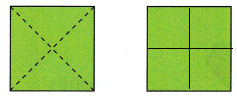Practice questions available in McGraw Hill Math Grade 2 Answer Key PDF Chapter 7 Test will engage students and is a great way of informal assessment.

Circle the name of the flat shape.

Question 1.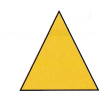hexagon
pentagon
triangleExplanation:
The above given shape is a triangle
So, i circled the word triangle.

Question 2.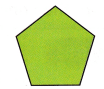triangle
cube
pentagon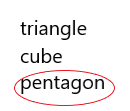Explanation:
The above shape is a pentagon
So, i circled the word pentagon.

Question 3.
Draw the shape that matches the clues. A shape with four sides. All sides are the same length.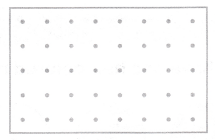Explanation:
I drew a shape based on the given clue
A square has 4 sides and all its sides are of same length
So, i drew a square.

Question 4.
Which shape is a cube? Complete the drawing of the cube.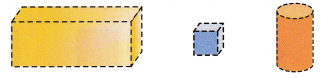Explanation:
I completed the drawing of the cube among all the shapes given above.

Question 5.
Draw lines. Divide the rectangle into 10 squares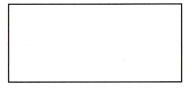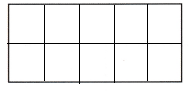Explanation:
I drew lines to divide the rectangle into 10 squares.

Question 6.
Draw lines to show fourths. Color one fourth of the rectangle green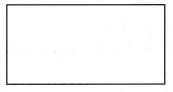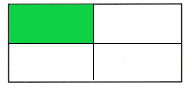Explanation:
I drew lines to show fourths and colored one fourth of the rectangle green.

Question 7.
How much of the rectangle is orange? Circle the answers.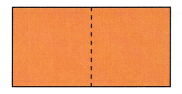one third
two halves
one wholeExplanation:
The rectangle is divided into 2 halves
So, it is colored one whole or two halves orange.

Question 8.
Draw lines to show thirds. Color one third of the circle.Explanation:
I drew 3 lines in the circle to show thirds
I colored one third of the circle.

Question 9.
What is another way to say one whole circle? Circle your answer.
two halves
two fourths
two thirds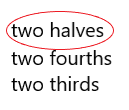Explanation:
Two halves is another way to say one whole circle
So, i drew a circle around the word two halves.

Question 10.
Show another way to make fourths.